# Newton's Second Law Of Motion

Newton’s second law of motion, unlike the first law of motion, pertains to the behaviour of objects for which all existing forces are unbalanced. The second law of motion is more quantitative and is used extensively to calculate what happens in situations involving a force. This article discusses Newton’s second law in detail.

## What is Newton’s Second Law of Motion?

Force is equal to the rate of change of momentum. For a constant mass, force equals mass times acceleration.Sir Issac newton

## Defining Newton’s Second Law of Motion

Newton’s second law states that the acceleration of an object depends upon two variables – the net force acting on the object and the mass of the object.  The acceleration of the body is directly proportional to the net force acting on the body and inversely proportional to the mass of the body. This means that as the force acting upon an object is increased, the acceleration of the object is increased. Likewise, as the mass of an object is increased, the acceleration of the object is decreased.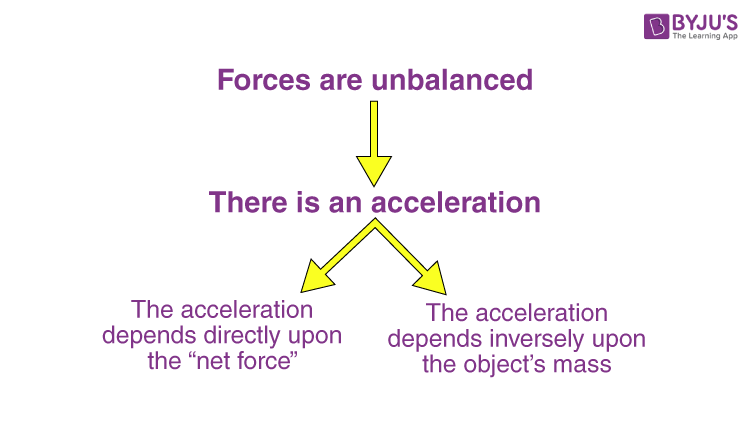Newton’s second law can be formally stated as,

The acceleration of an object as produced by a net force is directly proportional to the magnitude of the net force, in the same direction as the net force, and inversely proportional to the mass of the object.

This statement is expressed in equation form as,

$$\begin{array}{l}a=\frac{F_{net}}{m}\end{array}$$
The above equation can be rearranged to a familiar form as

$$\begin{array}{l}F=ma\end{array}$$
Since force is a vector, Newton’s second law can be written as

$$\begin{array}{l}\vec{F}=m\vec{a}\end{array}$$
The equation shows that the direction of the total acceleration vector points in the same direction as the net force vector.

## Deriving Newton’s Second Law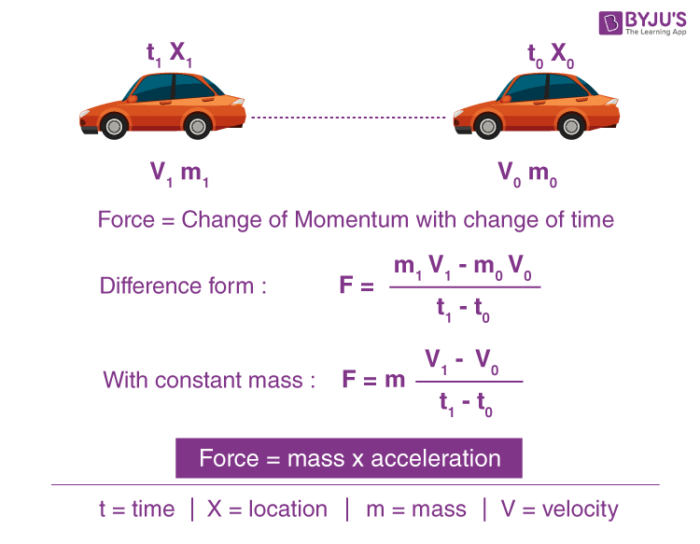### For Changing Mass

Let us assume that we have a car at a point (0) defined by location X0 and time t0. The car has a mass m0 and travels with a velocity v0. After being subjected to a force F, the car moves to point 1 which is defined by location X1 and time t1. The mass and velocity of the car change during the travel to values m1 and v1. Newton’s second law helps us determine the new values of m1 and v1 if we know the value of the acting force.

Taking the difference between point 1 and point 0, we get an equation for the force acting on the car as follows:

$$\begin{array}{l}F=\frac{m_1v_1-m_0v_0}{t_1-t_0}\end{array}$$

Let us assume the mass to be constant. This assumption is good for a car because the only change in mass would be the fuel burned between point “1” and point “0”. The weight of the fuel is probably small relative to the rest of the car, especially if we only look at small changes in time. Meanwhile, if we were discussing the flight of a bottle rocket, then the mass does not remain constant, and we can only look at changes in momentum.

### For Constant Mass

For a constant mass, Newton’s second law can be equated as follows:

$$\begin{array}{l}F=m\frac{v_1-v_0}{t_1-t_0}\end{array}$$
We know that acceleration is defined as the change in velocity divided by the change in time.
The second law then reduces to a more familiar form as follows:

$$\begin{array}{l}F=ma\end{array}$$
The above equation tells us that an object will accelerate if it is subjected to an external force. The amount of force is directly proportional to the acceleration and inversely proportional to the object’s mass.

 What is a Net Force? A net force ΣF is the sum of all forces acting on a body. More precisely, it is the vector sum of all forces acting on a body.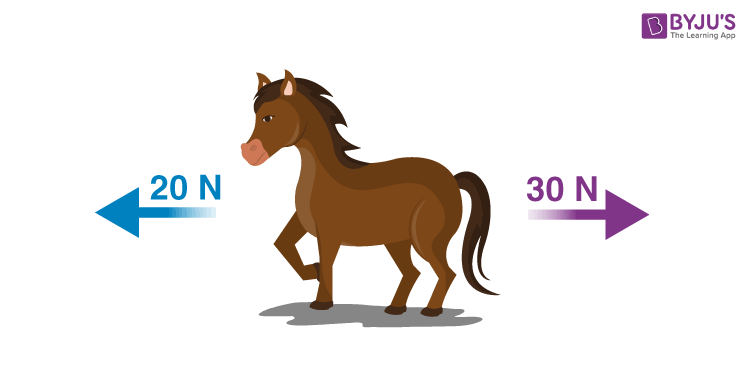Consider two forces of magnitude 30 N and 20 N that are exerted to the right and left, respectively on the horse shown in the picture. If we assume the rightward direction as positive, then the net force on the horse can be calculated as follows:   $$\begin{array}{l}\sum F=30\,N-20\,N=10\,N\end{array}$$   $$\begin{array}{l}\sum F=10\,N\,to \,the\, right\end{array}$$

## Application of Second Law

Newton’s second law is applied to identify the amount of force needed to make an object move or make it stop. Following are a few examples that we have listed to help you understand this point:

### Kicking a ball

When we kick a ball, we exert force in a specific direction. The stronger the ball is kicked, the stronger the force we put on it and the further away it will travel.

### Pushing a cart

It is easier to push an empty cart in a supermarket than a loaded one, and more mass requires more acceleration.

### Two people walking

Among the two people walking, if one is heavier than the other, the one weighing heavier will walk slower because the acceleration of the person weighing lighter is greater.

Get a glimpse of Newton’s second law of motion being taught in BYJU’S classes.## Newton’s Second Law Solved Examples

### Example 1:

If there is a block of mass 2kg, and a force of 20 N is acting on it in the positive x-direction, and a force of 30 N in the negative x-direction, then what would be its acceleration?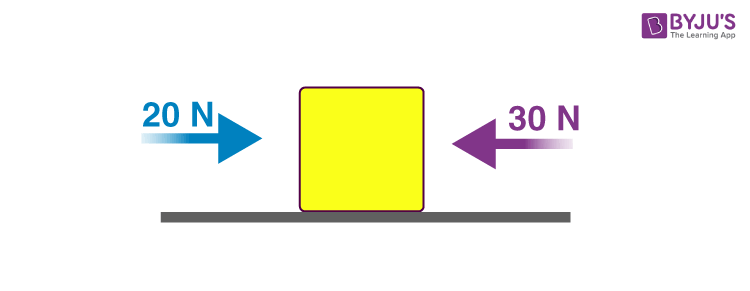We first have to calculate the net force acting on it to calculate its acceleration.

$$\begin{array}{l}F_{net} = 20 N – 30 N = -10 N\end{array}$$

Mass = 2kg

$$\begin{array}{l} Acceleration=\frac{-10\,N}{2\,kg}=- 5 m/s^2\end{array}$$

The negative acceleration indicates that the block is slowing and its acceleration vector is moving in an opposite direction directed opposite to the direction of motion.

### Example 2:

How much horizontal net force is required to accelerate a 1000 kg car at 4 m/s2?
Solution:
Newton’s 2nd Law relates an object’s mass, the net force on it, and its acceleration:
Therefore, we can find the force as follows:
Fnet = ma
Substituting the values, we get
1000 kg × 4 m/s2 = 4000 N
Therefore, the horizontal net force is required to accelerate a 1000 kg car at 4 m/s-2 is 4000 N.

Newton’s second law is applied in daily life to a great extent. For instance, in Formula One racing, the engineers try to keep the mass of cars as low as possible. Low mass will imply more acceleration, and the more the acceleration, the chances to win the race are higher.

## Top 10 Most Important and Expected Questions on Laws of Motion.## Frequently Asked Questions – FAQs

Q1

### How does Newton’s second law of motion apply to rockets?

According to Newton’s second law of motion, we know that force is a product of mass and acceleration. When a force is applied to the rocket, the force is termed as thrust. The greater the thrust, the greater will be the acceleration. Acceleration is also dependent on the rocket’s mass, and the lighter the rocket faster is the acceleration.

Q2

### How does Newton’s second law apply to a car crash?

According to the definition of Newton’s second law of motion, force is the dot product of mass and acceleration. The force in a car crash is dependent either on the mass or the acceleration of the car. As the acceleration or mass of the car increases, the force with which a car crash takes place will also increase.

Q3

### What is the other name for Newton’s second law?

The other name for Newton’s second law is the law of force and acceleration.
Q4

### What are some daily life examples of Newton’s second law of motion?

Newton’s second law of motion explains how force can change the acceleration of the object and how the acceleration and mass of the same object are related. Therefore, in daily life, if there is any change in the object’s acceleration due to the applied force, they are examples of Newton’s second law.
• Acceleration of the rocket is due to the force applied, known as thrust, and is an example of Newton’s second law of motion.
• Another example of Newton’s second law is when an object falls from a certain height, the acceleration increases because of the gravitational force.
Q5

### Write the formula for Newton’s second law of motion?

The formula for Newton’s second law of motion is F=ma.
Q6

### State Newton’s second law of motion

Newton’s second law of motion states that “Force is equal to the rate of change of momentum. For a constant mass, force equals mass times acceleration.
Q7

### For a constant mass, how is Newton’s second law equated?

For a constant mass, Newton’s second law can be equated as:
$$\begin{array}{l}F=m\frac{v_1-v_0}{t_1-t_0}\end{array}$$
.
Q8

### Define net force.

A net force ΣF is the sum of all forces acting on a body.
Q9

### State true or false:Net force is the vector sum of all forces acting on a body.

True.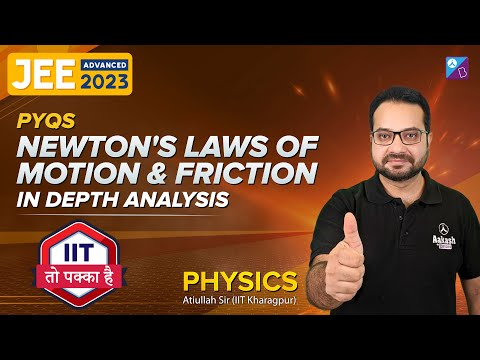Stay tuned to BYJU’S and KEEP FALLING IN LOVE WITH LEARNING!!

Test your Knowledge on Newtons Second Law Of Motion And Momentum

1. Super

2. Very nice explain

3. Superb explanation,understood the second law very well,prefer everyone to take byjus

4. perfect explanation

5. Very nice explanation 👍
I have understood very fastly
Thank you
BYJU’S

6. It’s very well explained but would prefer more examples/questions.

7. Very nice explanation. Thank you byjus

8. Beautiful explanation by India’s top teachers

9. Super BYJU’S